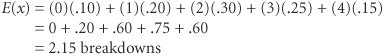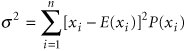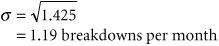# Expected Value[Page 490 ( continued )]

It is often possible to assign numeric values to the various outcomes that can result from an experiment. When the values of the variables occur in no particular order or sequence, the variables are referred to as random variables . Every possible value of a variable has a probability of occurrence associated with it. For example, if a coin is tossed three times, the number of heads obtained is a random variable. The possible values of the random variable are 0, 1, 2, and 3 heads. The values of the variable are random because there is no way of predicting which value (0, 1, 2, or 3) will result when the coin is tossed three times. If three tosses are made several times, the values (i.e., numbers of heads) that will result will have no sequence or pattern; they will be random.

Like the variables defined in previous chapters in this text, random variables are typically represented symbolically by a letter, such as x , y , or z . Consider a vendor who sells hot dogs outside a building every day. If the number of hot dogs the vendor sells is defined as the random variable x , then x will equal 0, 1, 2, 3, 4, . . . hot dogs sold daily.

Although the exact values of the random variables in the foregoing examples are not known prior to the event, it is possible to assign a probability to the occurrence of the possible values that can result. Consider a production operation in which a machine breaks down periodically. From experience it has been determined that the machine will break down 0, 1, 2, 3, or 4 times per month. Although managers do not know the exact number of breakdowns ( x ) that will occur each month, they can determine the relative frequency probability of each number of breakdowns P ( x ). These probabilities are as follows :

[Page 491]

x

P(x)

.10

1

.20

2

.30

3

.25

4

.15

1.00

These probability values taken together form a probability distribution . That is, the probabilities are distributed over the range of possible values of the random variable x .

The expected value of the random variable (the number of breakdowns in any given month) is computed by multiplying each value of the random variable by its probability of occurrence and summing these products.

The expected value of a random variable is computed by multiplying each possible value of the variable by its probability and summing these products .

For our example, the expected number of breakdowns per month is computed as follows:This means that, on the average, management can expect 2.15 breakdowns every month.

The expected value is often referred to as the weighted average, or mean , of the probability distribution and is a measure of central tendency of the distribution. In addition to knowing the mean, it is often desirable to know how the values are dispersed (or scattered ) around the mean. A measure of dispersion is the variance , which is computed as follows:

 1 Square the difference between each value and the expected value. 2 Multiply these resulting amounts by the probability of each value. 3 Sum the values compiled in step 2.

The expected value is the mean of the probability distribution of the random variable .

Variance is a measure of the dispersion of random variable values about the expected value, or mean .

The general formula for computing the variance, which we will designate as s 2 , isThe variance ( s 2 ) for the machine breakdown example is computed as follows:

x i

P(x i )

x i E(x)

[x i E(x)] 2

[x i E(x)] 2 P(x i )

.10

2.15

4.62

.462

1

.20

1.15

1.32

.264

2

.30

0.15

.02

.006

3

.25

0.85

.72

.180

4

.15

1.85

3.42

.513

1.00

1.425

s 2 = 1.425 breakdowns per month

The standard deviation is another widely recognized measure of dispersion. It is designated symbolically as s and is computed by taking the square root of the variance, as follows:The standard deviation is computed by taking the square root of the variance .

[Page 492]

## Management Science Application: A Probability Model for Analyzing Coast Guard Patrol Effectiveness

A primary responsibility of the U.S. Coast Guard is to monitor and protect the maritime borders of the United States. One measure of Coast Guard effectiveness in meeting this responsibility is determined by a probability model that indicates the probability that the Coast Guard will intercept a randomly selected target vessel attempting to penetrate a Coast Guard patrol perimeter. The probability P (I), the probability of intercepting the target vessel, is computed according to the formula

P (I) = P (ID) P (DA) P (AO) P (O)

P (ID) is the probability that a target vessel will be intercepted (I), given that it is detected (D). It is a constant value estimated from Coast Guard historical data. P (DA) is the probability that a target vessel is detected, given that a Coast Guard vessel is available (A). This value is computed using a submodel based on the patrol area and speed of the Coast Guard vessel and the speed of the target vessel. P (AO) is the probability that the Coast Guard vessel is available, given that it is on the scene (O) in the patrol area. P (O) is the probability that a Coast Guard vessel is on the scene in the patrol area, and currently it is assigned a constant value of one. The Coast Guard used this model to perform sensitivity analysis and analyze the effect of different parameter changes on effectiveness. An example might be testing the impact of a change in the patrol area or vessel speed.Source: S. O. Kimbrough, J. R. Oliver, and C. W. Pritchett, "On Post-Evaluation Analysis: Candle-Lighting and Surrogate Models," Interfaces 23, no. 3 (MayJune 1993): 1728.

A small standard deviation or variance, relative to the expected value, or mean, indicates that most of the values of the random variable distribution are bunched close to the expected value. Conversely, a large relative value for the measures of dispersion indicates that the values of the random variable are widely dispersed from the expected value.Introduction to Management Science (10th Edition)
ISBN: 0136064361
EAN: 2147483647
Year: 2006
Pages: 358

Similar book on Amazon

flylib.com © 2008-2017.
If you may any questions please contact us: flylib@qtcs.net